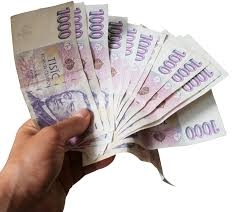# Procedure 34191

Petr got a fifth more than Pavel, Jirka got 100 CZK more than Petr, and Honza got half of what Petr did, so they shared the reward of CZK 2,500. Solution and procedure.

a =  600
h =  360
j =  820
p =  720

### Step-by-step explanation:

p+j+a+h=2500
p = a + a/5
j= 100+ p
h = p/2

a+h+j+p = 2500
6a-5p = 0
j-p = 100
2h-p = 0

Pivot: Row 1 ↔ Row 2
6a-5p = 0
a+h+j+p = 2500
j-p = 100
2h-p = 0

Row 2 - 1/6 · Row 1 → Row 2
6a-5p = 0
h+j+1.8333p = 2500
j-p = 100
2h-p = 0

Pivot: Row 2 ↔ Row 4
6a-5p = 0
2h-p = 0
j-p = 100
h+j+1.8333p = 2500

Row 4 - 1/2 · Row 2 → Row 4
6a-5p = 0
2h-p = 0
j-p = 100
j+2.3333p = 2500

Row 4 - Row 3 → Row 4
6a-5p = 0
2h-p = 0
j-p = 100
3.3333p = 2400

p = 2400/3.33333333 = 720
j = 100+p = 100+720 = 820
h = 0+p/2 = 0+720/2 = 360
a = 0+5p/6 = 0+5 · 720/6 = 600

a = 600
h = 360
j = 820
p = 720

Our linear equations calculator calculates it.Did you find an error or inaccuracy? Feel free to write us. Thank you!

Tips for related online calculators
Need help calculating sum, simplifying, or multiplying fractions? Try our fraction calculator.
Do you have a system of equations and looking for calculator system of linear equations?